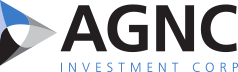Print Page     Close Window

SEC Filings

 10-Q AGNC INVESTMENT CORP. filed this Form 10-Q on 11/06/2015 Entire Document

_______________________
* Except as noted below, average numbers for each period are weighted based on days on our books and records. All percentages are annualized.

 1. Net asset value per common share is calculated as our total stockholders' equity, less our Series A and Series B Preferred Stock aggregate liquidation preference, divided by our number of common shares outstanding as of period end.
 2. We voluntarily discontinued hedge accounting for our interest rate swaps as of September 30, 2011. Please refer to our Interest Expense and Cost of Funds discussion further below and Notes 3 and 6 of our Consolidated Financial Statements in this Quarterly Report on Form 10-Q for additional information regarding our discontinuance of hedge accounting.
 3. Agency borrowings include agency repo, FHLB advances and debt of consolidated VIEs. Excludes U.S. Treasury repo agreements.
 4. Average stockholders' equity calculated as our average month-end stockholders' equity during the period.
 5. Average coupon for the period was calculated by dividing our total coupon (or cash) interest income on agency securities by our average agency securities held at par.
 6. Average asset yield for the period was calculated by dividing our total cash interest income on agency securities, adjusted for amortization of premiums and discounts, by our average amortized cost of agency securities held.
 7. Average cost of funds includes agency borrowings and interest rate swaps currently in effect, but excludes interest rate swap termination fees, forward starting swaps and costs associated with other supplemental hedges such as interest rate swaptions and short U.S. Treasury or TBA positions. Average cost of funds for the period was calculated by dividing our total cost of funds by our average agency borrowings outstanding for the period.
 8. TBA dollar roll income / (loss) is net of short TBAs used for hedging purposes. Dollar roll income excludes the impact of other supplemental hedges, and is recognized in gain (loss) on derivative instruments and other securities, net.
 9. Average cost of funds as of period end includes agency borrowings outstanding, plus the impact of interest rate swaps in effect as of each period end and forward starting swaps becoming effective, net of swaps expiring, within three months of each period end, but excludes costs associated with other supplemental hedges such as swaptions, U.S. Treasuries and TBA positions.
 10. Net comprehensive income (loss) return on average common equity for the period was calculated by dividing our comprehensive income/(loss) available /(attributable) to common stockholders by our average stockholders' equity, net of the Series A and Series B Preferred Stock aggregate liquidation preference.
 11. Economic return (loss) on common equity represents the sum of the change in our net asset value per common share and our dividends declared on common stock during the period over our beginning net asset value per common share.
 12. Average leverage during the period was calculated by dividing our daily weighted average agency borrowings outstanding for the period by the sum of our average stockholders' equity less our average investment in REIT equity securities for the period. Leverage excludes U.S. Treasury repurchase agreements.
 13. Average "at risk" leverage, inclusive of net TBA portfolio, during the period includes the components of "average leverage" plus our daily weighted average net TBA dollar position (at cost) during the period.
 14. Leverage at period end is calculated by dividing the sum of our agency borrowings outstanding and our receivable / payable for unsettled agency securities by the sum of our total stockholders' equity less the fair value of investments in REIT equity securities at period end. Leverage excludes U.S. Treasury repurchase agreements.
 15. "At risk" leverage at period end, inclusive of net TBA position, includes the components of "leverage (as of period end)" plus our net TBA dollar roll position outstanding as of period end, at cost.

Interest Income and Asset Yield
The following table summarizes our interest income for the three and nine months ended September 30, 2015 and 2014 (dollars in millions):
 Three Months Ended September 30, Nine Months Ended September 30, 2015 2014 2015 2014 Amount Yield Amount Yield Amount Yield Amount Yield Cash/coupon interest income \$ 423 3.62 % \$ 458 3.63 % \$ 1,422 3.62 % \$ 1,493 3.62 % Premium amortization (128 ) (1.20 )% (101 ) (0.92 )% (330 ) (0.96 )% (352 ) (0.97 )% Interest income \$ 295 2.42 % \$ 357 2.71 % \$ 1,092 2.66 % \$ 1,141 2.65 % Actual portfolio CPR 12 % 10 % 11 % 9 % Projected life CPR as of period end 9 % 8 % 9 % 8 % Average 30-year fixed rate mortgage rate as of period end 1 3.85 % 4.19 % 3.85 % 4.19 % 10-year U.S. Treasury rate as of period end 2.06 % 2.49 % 2.06 % 2.49 %
_______________________
 1. Source: Freddie Mac Primary Fixed Mortgage Rate Mortgage Market Survey

The principal elements impacting our interest income are the size of our average agency MBS portfolio and the yield on our investments. The following is a summary of the estimated impact of each of these elements on the increase in interest income for the three and nine months ended September 30, 2015 and 2014 (in millions):

38